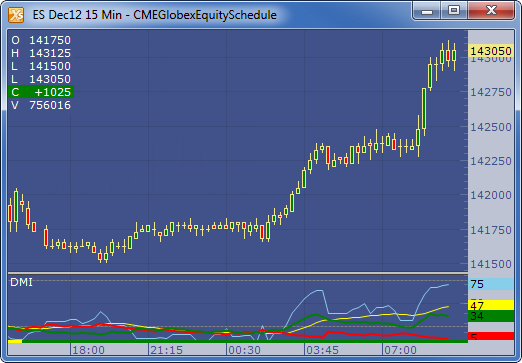← Back to X_TRADER® Help Library

X_STUDY® Documentation

Directional Movement Indicators (DMI)

Description

The Directional Movement Indicators (DMI) are components of the Directional Movement system published by J. Welles Wilder, and are computed with the Average Directional Movement Index (ADX). Two indicators are plotted, a Positive DI ( +DI ) and a Negative DI ( -DI ).

Formula

Directional Movement (DM) is defined as the largest part of the current period’s price range that lies outside the previous period’s price range. For each period calculate:

+DM =  positive or plus DM = High - Previous High

-DM = negative or minus DM = Previous Low - Low

The smaller of the two values is reset to zero, i.e., if +DM > -DM, then -DM = 0. On an inside bar (a lower high and higher low), both +DM and -DM are negative values, so both get reset to zero as there was no directional movement for that period.

The True Range (TR) is calculated for each period, where:

TR = Max of ( High - Low ), ( High -PreviousClose ), ( PreviousClose - Low )

The +DM, -DM and TR are each accumulated and smoothed using a custom smoothing method proposed by Wilder. For an n period smoothing, 1/n of each period’s value is added to the total each period, similar to an exponential smoothing:

+DMt = (+DMt-1 - (+DMt-1 / n))  + (+DMt)

-DMt = (-DMt-1 - (-DMt-1 / n)) + (-DMt)

TRt = (TRt-1 - (TRt-1 / n)) + (TRt)

Compute the positive/negative Directional Indexes, +DI and -DI, as a percentage of the True Range:

+DI = ( +DM / TR ) * 100

-DI = ( -DM / TR ) * 100

Example